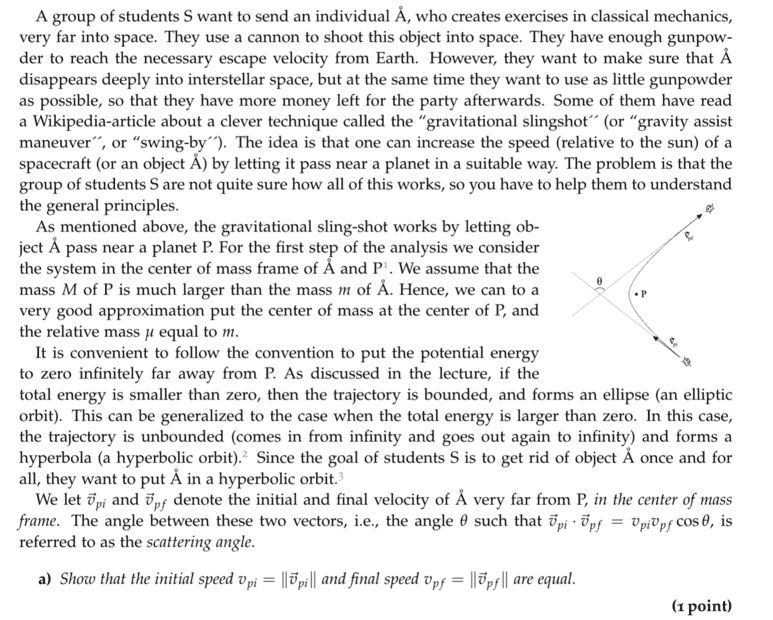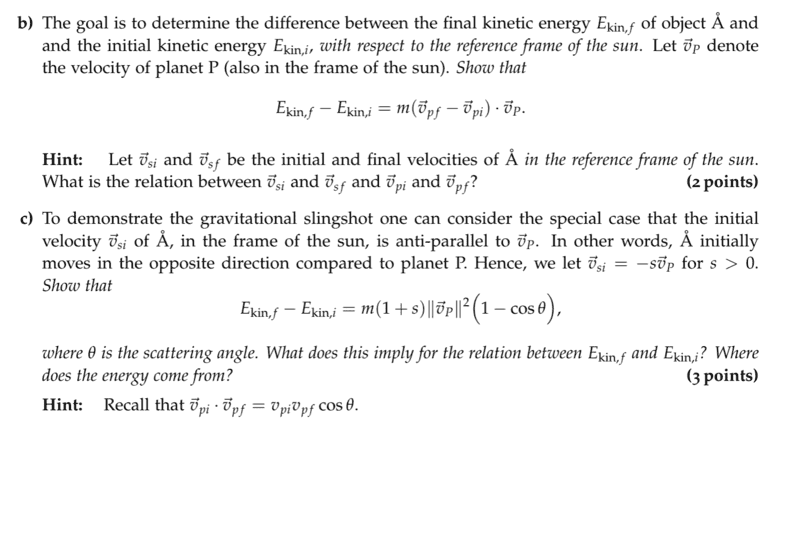# Solving a Celestial Mechanics Task with Velocity Vector Scattering

• Lambda96
In summary, the task is to find the change in kinetic energy of a particle that is scattered by an angle from its original direction. The change in kinetic energy is expressed in terms of the initial and final velocities relative to the planet.f

#### Lambda96

Homework Statement
Find the difference in kinetic energy when the velocities of the object and planet are antiparallel
Relevant Equations
none
Hi,Unfortunately, I am not getting anywhere at all with task c. I have now proceeded as follows:

I assume that the calculation takes place in the reference system of the sun. In the task the following is valid, $$\vec{v}_{si}=-s\vec{v}_p$$ I have now simply assumed that the planet is scattered the velocity vector by the angle $$\theta$$ so the following is valid for the final velocity

$$\vec{v}_{sf}=-s\vec{v}_p*cos(\theta)$$

After that I simply made the difference in the kinetic energy, i.e.

$$E_{kin,f}-E_{kin,i}=\frac{1}{2}m\vec{v}_{sf}^2 - \frac{1}{2}m\vec{v}_{si}^2$$

If I now insert $$\vec{v}_{sf}=-s\vec{v}_p*cos(\theta)$$ and $$\vec{v}_{si}=-s\vec{v}_p$$, I immediately see that I do not get the required equation, since I have terms like $$s^2$$ and $$cos(\theta)^2$$. But I also don't understand how to get this equation where $$s$$ and $$cos(\theta)$$ occur, you have to square the velocity for the kinetic energy.

I assume that the calculation takes place in the reference system of the sun.
Part (b) is setting you up for part (c). Thus, you can express the change in KE of ##\mathrm{\mathring{A}}## relative to the sun in terms of the initial and final velocities of ##\mathrm{\mathring{A}}## relative to the planet P! This makes things easier because the initial and final velocities relative to P have a simple relationship (as shown in part (a)).

In the task the following is valid, $$\vec{v}_{si}=-s\vec{v}_p$$
OK. Can you use this to find an expression for ##\vec{v}_{pi}## in terms of ##s## and ##\vec{v}_P##?

I have now simply assumed that the planet is scattered the velocity vector by the angle $$\theta$$ so the following is valid for the final velocity

$$\vec{v}_{sf}=-s\vec{v}_p*cos(\theta)$$
This equation can't be right. The right-hand side represents a vector quantity that has a direction either parallel or antiparallel to ##\vec{v}_P## (depending on the sign of ##\cos \theta##). But that's certainly not the right direction for ##\vec{v}_{sf}##. However, if you use the expression for the change in KE as given in part (b), you don't need ##\vec{v}_{sf}##. Instead, you need ##\vec{v}_{pf}##. Or, more specifically, you need ##\vec{v}_{pf} \cdot \vec{v}_P##.

Note that ##\mathrm{\mathring{A}}## is scattered by the angle ##\theta## in the frame of reference of the planet, not in the frame of reference of the sun.

Last edited:
•jim mcnamara
Thanks for your help TSny, with your help I was able to solve the task of•TSny